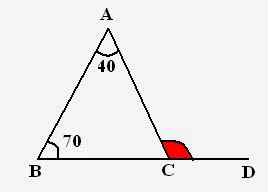Emily-Jane Bray

2021-02-26

Divisibility Rules: Quantitative Aptitude Section There are some specific rules by which we can determine the divisor of the given number. Today I will discuss divisibility rules from 2 to 19. Using these rules you can easily determine a divisor of given number, however large it may be. Let me tell you the rules of divisibility from 2 to 19.## Triangles

Triangle = Figure with three sides.  Study the following triangle: A,B,C to points. a, b, c to sides. x, y, z to angles.Perimeter of triangle = a + b + c
Remember that, Sum of all the angles is always ${180}^{\circ }$
i.e. x + y + z = ${180}^{\circ }$

### Classification of Triangles

Basically there are three types of triangles excluding right angle triangle. Let me tell you how they vary from each other.

• Scalene Triangle
• Isosceles Triangle
• Equilateral Triangle

Scalene Triangle: No side of triangle is equal.
Isosceles Triangle: Two sides of triangle are equal.
Equilateral Triangle: All sides of triangle are equal.

 Scalene Isosceles Equilateral Definition a ne b ne c ane b = c a = b = c Area A $\left(b/4\right)\sqrt{4{a}^{2}–{b}^{2}}$ $\left(3{\right)}^{\left(1/2\right)\left({a}^{2}\right)/4}$ Height - $\sqrt{\left(4{a}^{2}-{b}^{2}\right)/2}$ $\left(3{\right)}^{\left(1/2\right)a/2}$

$A={s\left(s-a\right)\left(s-b\right)\left(s-c\right)}^{1/2}$
where, s = (a+ b+ c)/2

### Properties of external angles of Triangle:

1. Sum of all exterior angles is ${360}^{\circ }$
Study the following set of triangles and their exterior angles,a, b, c to Interior angles. p, q, r and s, t, u to Exterior angles.
So, sum of exterior angles = ${360}^{o}$ i.e. p + q + r = 360^o and s + t + u = ${360}^{o}$
2. Next property of exterior angle which is important in paper point of view:

External angle = Sum of two internal angles.

For example: In above figures,
r = a + b
q = a + c
s = b + c and so on.

## Right angle Triangle

Following triangle is a right angle triangle i.e. a triangle with one out of three 90^o angle.### Area of right angle triangle

Area = 1/2 times Base times Perpendicular

### Example with Solution

Example: In following figure, CE is perpendicular to AB, angle ACE = 20^o and angle ABD = 50^o. Find angle BDA:Solution: To Find: angle BDA
For this what we need --- angle BAD  Because, Sum of all angles = ${180}^{o}$
Consider, triangle ECA,
CEA + EAC + ACE = ${180}^{o}$ i.e. ${90}^{o}$${20}^{o}$ + EAC = ${180}^{o}$ Therefore, EAC = ${70}^{o}$
Now, come to triangle ABD,
ABD + BDA + BAD = ${180}^{o}$  ${70}^{o}$ + ${50}^{o}$ + BAD = ${180}^{o}$ Therefore, BAD = ${60}^{o}$
Example: In given figure. BC is produced to D and angle BAC = ${40}^{o}$ and angle ABC = ${70}^{o}$. Find angle ACD:Solution: In above figure, ACD is an exterior angle, and according to property, Exterior angle = Sum of interior angles Therefore, ACD = ${70}^{o}$ + ${40}^{o}$ =${110}^{o}$
This is not the end of this chapter. These are just the basics. In next session, I will discuss some important results, properties (congruency, similarity) and much more. Always remember, Geometry needs practice and time.

Do you have a similar question?